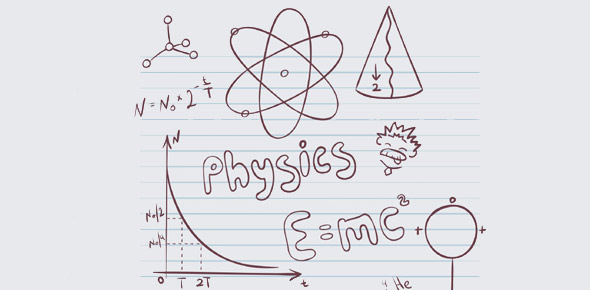# Physics Chapter 23

63 Questions | Total Attempts: 133SettingsPhysics Chapter 23

Related Topics
• 1.
Electrons are made to flow in a wire when there is
• A.

An imbalance of charges in the wire

• B.

More potential energy at one end of the wire than the other

• C.

A potential difference across its ends

• 2.
An ampere is a unit of electrical
• A.

Pressure

• B.

Current

• C.

Resistance

• D.

All of these

• E.

None of these

• 3.
A wire that carries an electric current
• A.

Is electrically charged

• B.

May be electrically charged

• C.

Is never electrically charged

• 4.
A coulomb of charge that passes through a 6-volt battery is given
• A.

6 joules

• B.

6 amperes

• C.

6 ohms

• D.

6 watts

• E.

6 newtons

• 5.
Which statement is correct?
• A.

Charge flows in a circuit

• B.

Voltage flows through a circuit

• C.

Resistance is established across a circuit

• D.

Current causes voltage

• 6.
Electrons move in an electrical circuit
• A.

By being bumped by other electrons

• B.

By colliding with molecules

• C.

By interacting with an established electric field

• D.

Because the wires are so thin

• E.

None of these

• 7.
Heat a copper wire and its electric resistance
• A.

Decreases

• B.

Remains uncharged

• C.

Increases

• 8.
Stretch a copper wire so that it is thinner and the resistance between its ends
• A.

Decreases

• B.

Remains unchanged

• C.

Increases

• 9.
A wire carrying a current is normally charged
• A.

Negatively

• B.

Positively

• C.

Not at all

• 10.
In an ac circuit, the electric field
• A.

Increases via the inverse square law

• B.

Changes magnitude and direction with time

• C.

Is everywhere the same

• D.

Is non-existent

• E.

None of these

• 11.
The current through a 10-ohm resistor connected to a 120-V power supply is
• A.

1 A

• B.

10 A

• C.

12 A

• D.

120 A

• E.

None of these

• 12.
A 10-ohm resistor has a 5-A current in it. What is the voltage across the resistor?
• A.

5 V

• B.

10 V

• C.

15 V

• D.

20 V

• E.

More than 20 V

• 13.
When a 10-V battery is connected to a resistor, the current in the resistor is 2A. What is the resistor's value?
• A.

2 ohms

• B.

5 ohms

• C.

10 ohms

• D.

20 ohms

• E.

More than 20 ohms

• 14.
The source of electrons in an ordinary electrical circuit is
• A.

A dry cell, wet cell or battery

• B.

The back emf of motors

• C.

The power station generator

• D.

The electrical conductor itself

• E.

None of these

• 15.
The source of electrons lighting an incandescent as light bulb is
• A.

The power company

• B.

Electrical outlet

• C.

Atoms in the light bulb filament

• D.

The wire leading to the lamp

• E.

The source voltage

• 16.
A woman experiences an electrical shock. The electrons making the shock come from the
• A.

Woman's body

• B.

Ground

• C.

Power plant

• D.

Hairdryer

• E.

Electric field in the air

• 17.
In a common dc circuit, electrons move at speeds of
• A.

A fraction of a centimeter per second

• B.

Many centimeters per second

• C.

The speed of a sound wave

• D.

The speed of light

• E.

None of these

• 18.
When a light switch is turned on in a dc circuit, the average speed of electrons in the lamp is
• A.

The speed of sound waves in metal

• B.

The speed of light

• C.

1000 cm/s

• D.

Less than 1 cm/s

• E.

Dependent on how quickly each electron bumps into the next electron

• 19.
Alternating current is normally produced by a
• A.

Battery

• B.

Generator

• C.

Both of these

• D.

Neither of these

• 20.
The electric power of a lamp that carries 2 A at 120 V is
• A.

1/6 watts

• B.

2 watts

• C.

60 watts

• D.

20 watts

• E.

240 watts

• 21.
When two lamps are connected in parallel to a battery, the electrical resistance that the battery senses is
• A.

More than the resistance of either lamp

• B.

Less than the resistance of either lamp

• C.

None of these

• 22.
When two lamps are connected in series to a battery, the electrical resistance that the battery senses is
• A.

More than the resistance of either lamp

• B.

Less than the resistance of either lap

• C.

None of these

• 23.
On some early automobiles both headlights went out when one bulb burned out. The headlights must have been connected in
• A.

Parallel

• B.

Perpendicular

• C.

Series

• D.

Haste

• 24.
Modern automobile headlights are connected in
• A.

Parallel

• B.

Perpendicular

• C.

Series

• D.

None of these

• 25.
There are electrons int he filament of the ac lamp in your bedroom. When you turn on the lamp and it glows, the glowing comes from
• A.

Different electrons; the ones that flow in the circuit to your lamp

• B.

The same electrons

• C.

The positive charges that flow in the filament